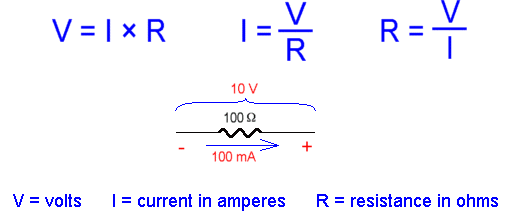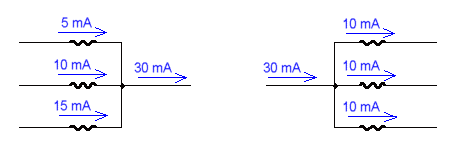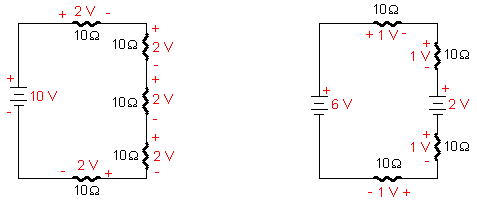Home > Electronic Tutorials > General Theory > Ohms & Kirchhoff's Law Tutorial

General Theory - Electronic Tutorials

# Ohms & Kirchhoff's Law Tutorial## Kirchhoff's Current Law

The algebraic sum of currents entering and leaving any point in a circuit must equal zero.

Stated another way

No matter how many paths into and out of a single point all the current leaving that point must equal the current arriving at that point.## Kirchhoff's Voltage Law

The algebraic sum of the voltages around any closed path is zero.

Stated another way

The voltage drops around any closed loop must equal the applied voltages.When voltages are opposing as seen at the right, the difference is the voltage applied to the circuit. In this case 4 volts must be dropped by the resistors to equal the applied voltage.

Note: To report broken links or to submit your projects please send email to Webmaster

 Discover